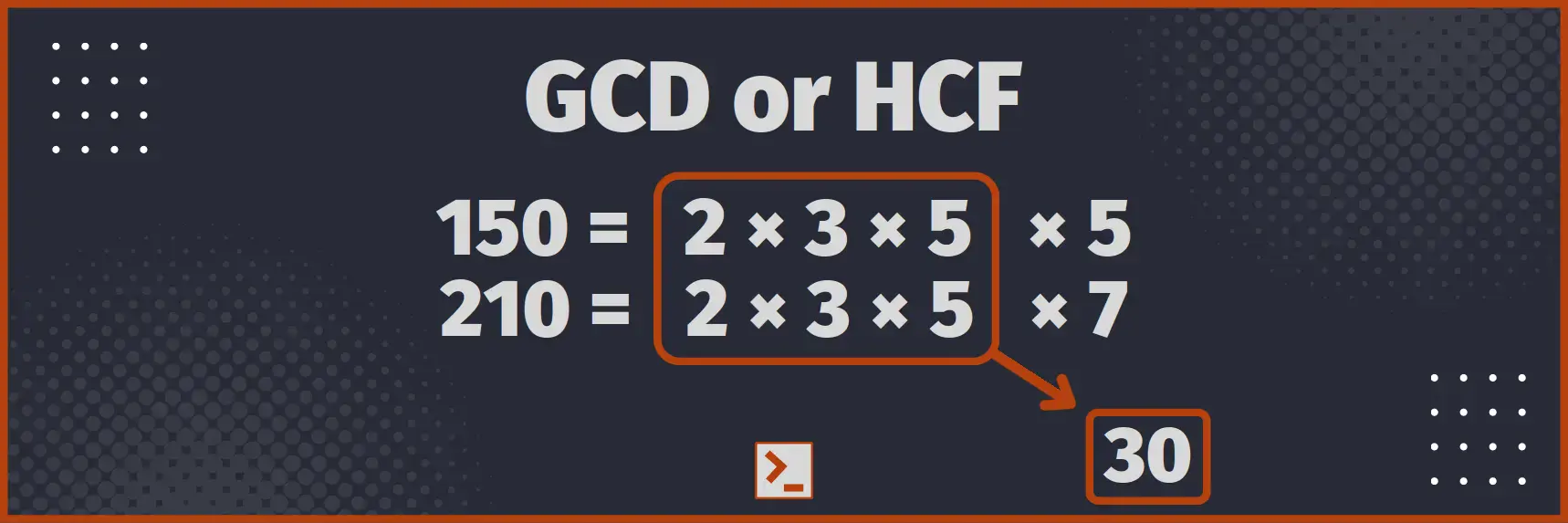# GCD or HCF of two numbers

and program in Rust to calculate it using Euclidean algorithm.

## What is GCD or HCF

Greatest Common Divisor ( GCD ) or Highest Common Factor ( HCF ) of two natural numbers is the largest natural number that divides both numbers.

We can also say it is the largest natural number that is factor of both numbers.

For Example : GCD of 100 and 75 is 25## Naive Approach

Let us suppose we have to find gcd or hcf of 2 numbers, a and b.

Naive or brute force approach is to traverse all the numbers from 1 to min(a, b), and check if the number divides both numbers. Function using this approach is

```fn gcd(a:usize, b:usize) -> usize{    // Initialize ans, or answer and limit    let mut ans:usize = 1;    let limit = min(a,b);
// Loop from 2 to limit, both inclusive    for i in 2..(limit+1) {        // Check if both a and b are divisible        if a%i == 0 && b%i == 0 {            ans = i;        }    }    return ans;}```

Program With driver code

```use std::cmp::min;use std::io::stdin;
fn take_vector() -> Vec<usize> {    let mut input = String::new();    stdin().read_line(&mut input).unwrap();    let arr: Vec<usize> = input.trim().        split_whitespace().map(|x| x.parse().unwrap()).collect();    return arr;}
// Magic Starts here
fn gcd(a:usize, b:usize) -> usize{    // Initialize ans, or answer and limit    let mut ans:usize = 1;    let limit = min(a,b);
// Loop from 2 to limit, both inclusive    for i in 2..(limit+1) {        // Check if both a and b are divisible        if a%i == 0 && b%i == 0 {            ans = i;        }    }    return ans;}
// Driver Code
pub fn main() {    let numbers = take_vector();    println!("{}", gcd(numbers, numbers));}```

Input

210 150

Output

30

Time Complexity : O( min (a, b) )
Space Complexity : O( 1 )

## Efficient Euclidean algorithm

We can find the gcd of 2 numbers using Euclidean algorithm in logarithmic time complexity

Refer Wikipedia

In simple words, let us suppose that we have to find gcd of 2 distinct numbers, a and b, such that a > b.

• If a%b == 0, then obviously b is the gcd.
• If a%b != 0, then gcd must divide both b and a%b ( from Euclidean algorithm or even general observation ). So, we can now find gcd of b and a%b, which will be the answer.

Also, clearly, b > a%b.

Function using this approach is

```fn gcd(mut a:usize, mut b:usize) -> usize{    // If equal, return any of them    if a==b {        return a;    }
// Swap a with b, if b is greater than a    if b > a {        std::mem::swap(&mut a, &mut b);    }
while b>0 {        // This is the trickiest part        // We swap a with b, and b with a%b, till b becomes 0        let temp = a;        a = b;        b = temp%b;    }
// Now, a%b = 0, hence return it    return a;}```

Use the same driver code.

Input

210 150

Output

30

Time Complexity : O( log(min (a, b)) )
Space Complexity : O( 1 )

## Conclusion

Greatest Common Divisor ( GCD ) or Highest Common Factor ( HCF ) of two natural numbers is the largest natural number that divides both numbers. In this article, we made a program to compute GCD or HCF in Logarithmic Time Complexity using Euclidean algorithm.

Here is the optimized function for easy access

`fn gcd(mut a:usize, mut b:usize) -> usize{    if a==b { return a; }    if b > a { std::mem::swap(&mut a, &mut b); }    while b>0 {        let temp = a;        a = b;        b = temp%b;    }    return a;}`

Thank You